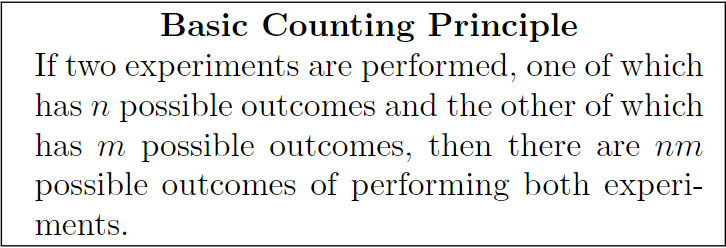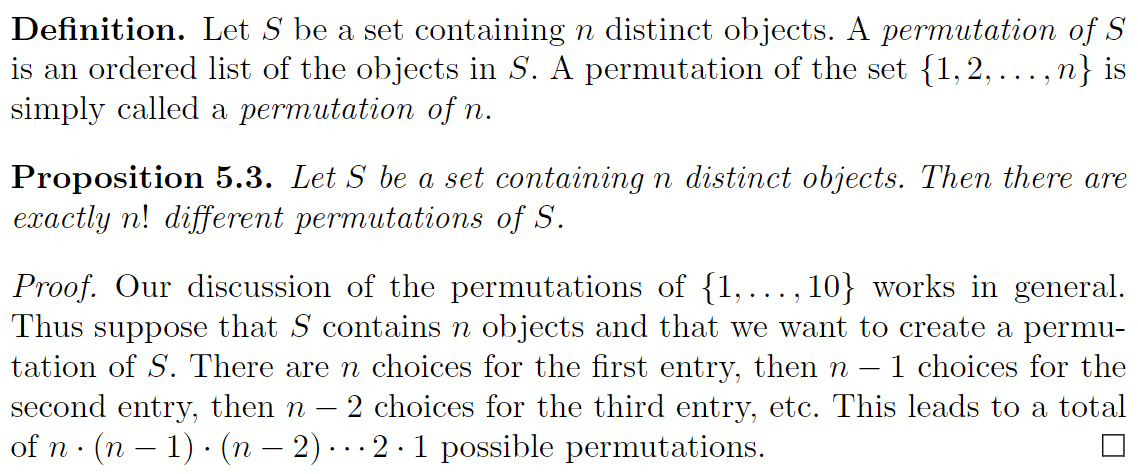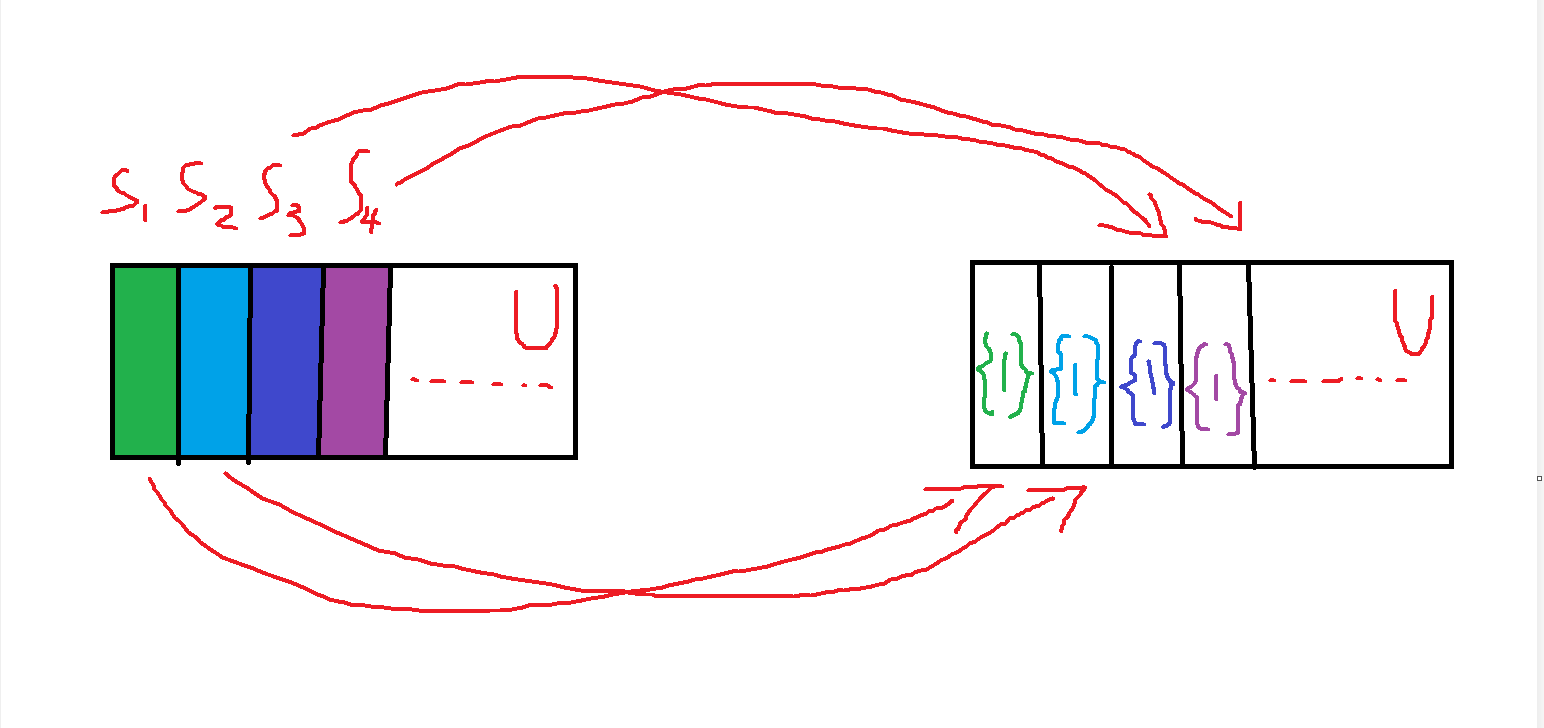# 1.组合数学

## 1.0.基本计数原理Example

Bob去一家餐馆吃饭，那里有两种前菜：鸡蛋卷和锅贴；以及20种主菜。Bob计划下单一种前菜和一种主菜，问题是：

Bob总共能下多少种可能的单？

(0,0),(0,1),…,(0,19),

(1,0),(1,1),…,(1,19)

2*20 = 40

## 1.1.(全)排列Example

S = {0,1,2,3,4,5,6,7,8,9}

$10! = 10*9*8...2*1 = 3628800$

$S_{10}^{10} = 10*9*8...2*1 = 10! = 3628800$

$S_N^n = (N-0)*(N-1)*...*(N-n+1)$

(在本文中我用 $S_N^n$ 表示从N个不同的元素中选取其中的n个进行排列)

S = {A,A,A,B,B}

$S_5^5 = 5! = 120$

$S_2^2 = 2! = 2$ 种排列方式

$\frac{S_5^5}{S_3^3*S_2^2} = 10$

（是否重复，很明显需要分类，所以这里用加法）

S = {A,A,A}

$S_3^3 \mapsto 1$

$f(S) = S*S^{-1}$

Input:S

Output: {1}

$f(A*B) = A*B*A^{-1}*B^{-1}$## 1.2.组合

Example

Alice先点，有20种选择，Bob再点，有剩下的19种选择……

$S_{20}^5 = 20*19*18*17*16 = 1860480$

E被映射成了S，其中 #E = 1 ， #S = 120

(我们用 #S 表示 S 这个集合中元素的总数)

$\binom{20}{5} = \frac{20*19*18*17*16}{5!} = 15504$

$\binom{n}{r} = \frac{(n-0)*(n-1)*...*(n-r+1)}{r!}$

$\binom{n}{r} = \frac{n!}{r!(n-r)!}$

## 1.3.二项式定理

$(x+y)^4 = ax^4 + bxy^3 + cx^2y^2 + dx^3y + ey^4$

$a,b,c,d,e$ 分别是多少？

$a = \binom{4}{4} = 1$

$b = \binom{4}{1}$

$c = \binom{4}{2}$

$d = \binom{4}{3}$

$e = \binom{4}{0}$